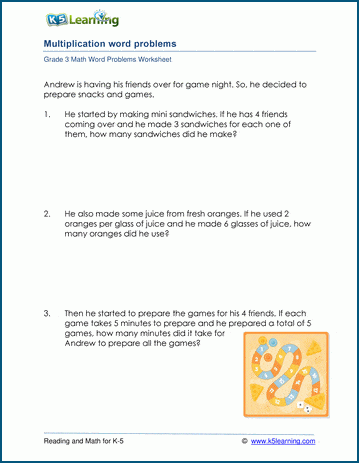# Multiplication Word Problems for 3rd Grade

## Simple third grade multiplication word problems

These worksheets contain simple multiplication word problems.  The student should read the word problem and derive a multiplication equation from it.  He or she can then solve the problem by mental multiplication and express the answer in the appropriate units.  Students should have a concrete understanding of the meaning of multiplication before attempting these worksheets

## Multiplication word problems with multiples of 10

These grade 3 math word problems can be solved by multiplying numbers by multiples of 10.  Most of these problems can be solved mentally after the student has derived the multiplication equation.

## Multiplication in columns word problems

These math word problems will require column form multiplication of varying difficulty.  Answers should be expressed in suitable units by the third grade student.  Students should be comfortable with column form multiplication before attempting these problems, as the focus should be on the application of mathematics to real world situations, rather than the mechanical techniques of multiplication.

## More grade 3 multiplication word problems

For extra practice, following are a mixture of supplementary third grade multiplication word problems, most requiring multiplication in columns.## Mixed multiplication & division word problems for grade 3

These math word problems may require multiplication or division to solve.  The student will be challenged to read the problem carefully, think about the true meaning of the situation to answer the question in a full sentence.  Attempt these problems after the student had become proficient at both multiplication and division word problems.Sample Grade 3 Multiplication Fraction Word Problem Worksheet

## More word problem worksheets

Explore all of our math word problem worksheets, from kindergarten through grade 5.

## What is K5?

K5 Learning offers reading and math worksheets, workbooks and an online reading and math program for kids in kindergarten to grade 5.  We help your children build good study habits and excel in school.